Warning: mysql_num_rows(): supplied argument is not a valid MySQL result resource in /home/virus/new/bbs/Sources/Security.php on line 67
Classic Texts: Bertrand Russell - Philosophy of Mathematical Logic (1911)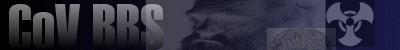Welcome, Guest. Please Login or Register. 2023-06-03 01:37:40 CoV Wiki Learn more about the Church of Virus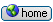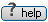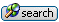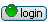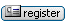News: Read the first edition of the Ideohazard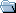Church of Virus BBS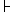General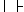Philosophy & Religion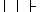Classic Texts: Bertrand Russell - Philosophy of Mathematical Logic (1911) « previous next »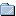Author Topic: Classic Texts: Bertrand Russell - Philosophy of Mathematical Logic (1911)  (Read 846 times)
rhinoceros
Archon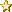Gender: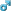Posts: 1318
Reputation: 8.14
Rate rhinocerosMy point is ...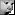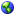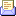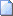Classic Texts: Bertrand Russell - Philosophy of Mathematical Logic (1911) « on: 2003-06-22 12:26:05 »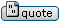Bertrand Russell

The Philosophical Importance of Mathematical Logic (1911)

Source: Collected Papers of Bertrand Russell (1972) publ. Routledge. Two articles reproduced here.IN SPEAKING OF "Mathematical logic", I use this word in a very broad sense. By it I understand the works of Cantor on transfinite numbers as well as the logical work of Frege and Peano. Weierstrass and his successors have "arithmetised" mathematics; that is to say, they have reduced the whole of analysis to the study of integer numbers. The accomplishment of this reduction indicated the completion of a very important stage, at the end of which the spirit of dissection might well be allowed a short rest. However, the theory of integer numbers cannot be constituted in an autonomous manner, especially when we take into account the likeness in properties of the finite and infinite numbers. It was, then, necessary to go farther and reduce arithmetic, and above all the definition of numbers, to logic. By the name "mathematical logic", then, I will denote any logical theory whose object is the analysis and deduction of arithmetic and geometry by means of concepts which belong evidently to logic. It is this modern tendency that I intend to discuss here.

In an examination of the work done by mathematical logic, we may consider either the mathematical results, the method of mathematical reasoning as revealed by modern work, or the intrinsic nature of mathematical propositions according to the analysis which mathematical logic makes of them. It is impossible to distinguish exactly these three aspects of the subject, but there is enough of a distinction to serve the purpose of a framework for discussion. It might be thought that the inverse order would be the best; that we ought first to consider what a mathematical proposition is, then the method by which such propositions are demonstrated, and finally the results to which this method leads us. But the problem which we have to resolve, like every truly philosophical problem, is a problem of analysis; and in problems of analysis the best method is that which sets out from results and arrives at the premises. In mathematical logic it is the conclusions which have the greatest degree of certainty: the nearer we get to the ultimate premises the more uncertainty and difficulty do we find.

From the philosophical point of view, the most brilliant results of the new method are the exact theories which we have been able to form about infinity and continuity. We know that when we have to do with infinite collections, for example the collection of finite integer numbers, it is possible to establish a one-to-one correspondence between the whole collection and a part of itself. For example, there is such a correspondence between the finite integers and the even numbers, since the relation of a finite number to its double is one-to-one. Thus it is evident that the number of an infinite collection is equal to the number of a part of this collection. It was formerly believed that this was a contradiction; even Leibnitz, although he was a partisan of the actual infinite, denied infinite number because of this supposed contradiction. But to demonstrate that there is a contradiction we must suppose that all numbers obey mathematical induction. To explain mathematical induction, let us call by the name "hereditary property" of a number a property which belongs to n + 1 whenever it belongs to n. Such is, for example, the property of being greater than 100. If a number is greater than 100, the next number after it is greater than 100. Let us call by the name "inductive property" of a number a hereditary property which is possessed by the number zero. Such a property must belong to 1, since it is hereditary and belongs to 0; in the same way, it must belong to 2, since it belongs to 1; and so on. Consequently the numbers of daily life possess every inductive property. Now, amongst the inductive properties of numbers is found the following. If any collection has the number n, no part of this collection can have the same number n. Consequently, if all numbers possess all inductive properties, there is a contradiction with the result that there are collections which have the same number as a part of themselves. This contradiction, however, ceases to subsist as soon as we admit that there are numbers which do not possess all inductive properties. And then it appears that there is no contradiction in infinite number. Cantor has even created a whole arithmetic of infinite numbers, and by means of this arithmetic he has completely resolved the former problems on the nature of the infinite which have disturbed philosophy since ancient times.

The problems of the continuum are closely connected with the problems of the infinite and their solution is effected by the same means. The paradoxes of Zeno the Eleatic and the difficulties in the analysis of space, of time, and of motion, are all completely explained by means of the modern theory of continuity. This is because a non-contradictory theory has been found, according to which the continuum is composed of an infinity of distinct elements; and this formerly appeared impossible. The elements cannot all be reached by continual dichotomy; but it does not follow that these elements do not exist.

From this follows a complete revolution in the philosophy of space and time. The realist theories which were believed to be contradictory are so no longer, and the idealist theories have lost any excuse there might have been for their existence. The flux, which was believed to be incapable of analysis into indivisible elements, shows itself to be capable of mathematical analysis, and our reason shows itself to be capable of giving an explanation of the physical world and of the sensible world without supposing jumps where there is continuity, and also without giving up the analysis into separate and indivisible elements.

The mathematical theory of motion and other continuous changes uses, besides the theories of infinite number and of the nature of the continuum, two correlative notions, that of a function and that of a variable. The importance of these ideas may be shown by an example. We still find in books of philosophy a statement of the law of causality in the form: "When the same cause happens again, the same effect will also happen." But it might be very justly remarked that the same cause never happens again. What actually takes place is that there is a constant relation between causes of a certain kind and the effects which result from them. Wherever there is such a constant relation, the effect is a function of the cause. By means of the constant relation we sum up in a single formula an infinity of causes and effects, and we avoid the worn-out hypothesis of the repetition of the same cause. It is the idea of functionality, that is to say the idea of constant relation, which gives the secret of the power of mathematics to deal simultaneously with an infinity of data.

To understand the part played by the idea of a function in mathematics, we must first of all understand the method of mathematical deduction. It will be admitted that mathematical demonstrations, even those which are performed by what is called mathematical induction, are always deductive. Now, in a deduction it almost always happens that the validity of the deduction does not depend on the subject spoken about, but only on the form of what is said about it. Take for example the classical argument: All men are mortal, Socrates is a man, therefore Socrates is mortal. Here it is evident that what is said remains true if Plato or Aristotle or anybody else is substituted for Socrates. We can, then, say: If all men are mortal, and if x is a man, then x is mortal. This is a first generalisation of the proposition from which we set out. But it is easy to go farther. In the deduction which has been stated, nothing depends on the fact that it is men and mortals which occupy our attention. If all the members of any class a are members of a class s, and if x is a member of the class a, then x is a member of the class s. In this statement, we have the pure logical form which underlies all the deductions of the same form as that which proves that Socrates is mortal. To obtain a proposition of pure mathematics (or of mathematical logic, which is the same thing), we must submit a deduction of any kind to a process analogous to that which we have just performed, that is to say, when an argument remains valid if one of its terms is changed, this term must be replaced by a variable, i.e. by an indeterminate object. In this way we finally reach a proposition of pure logic, that is to say a proposition which does not contain any other constant than logical constants. The definition of the logical constants is not easy, but this much may be said: A constant is logical if the propositions in which it is found still contain it when we try to replace it by a variable. More exactly, we may perhaps characterise the logical constants in the following manner: If we take any deduction and replace its terms by variables, it will happen, after a certain number of stages, that the constants which still remain in the deduction belong to a certain group, and, if we try to push generalisation still farther, there will always remain constants which belong to this same group. 'This group is the group of logical constants. The logical constants are those which constitute pure form; a formal proposition is a proposition which does not contain any other constants than logical constants. We have just reduced the deduction which proves that Socrates is mortal to the following form: "If x is an a, then, if all the members of a are members of b, it follows that x is a b." The constants here are: is-a, all, and if-then. These are logical constants and evidently they are purely formal concepts.

Now, the validity of any valid deduction depends on its form, and its form is obtained by replacing the terms of the deduction by variables, until there do not remain any other constants than those of logic. And conversely: every valid deduction can be obtained by starting from a deduction which operates on variables by means of logical constants, by attributing to variables definite values with which the hypothesis becomes true.

By means of this operation of generalisation, we separate the strictly deductive element in an argument from the element which depends on the particularity of what is spoken about. Pure mathematics concerns itself exclusively with the deductive element. We obtain propositions of pure mathematics by a process of purification. If I say: "Here are two things, and here are two other things, therefore here arc four things in all", I do not state a proposition of pure mathematics because here particular data come into question. The proposition that I have stated is an application of the general proposition: "Given any two things and also any two other things, there are four things in all." 'The latter proposition is a proposition of pure mathematics, while the former is a proposition of applied mathematics.

It is obvious that what depends on the particularity of the subject is the verification of the hypothesis, and this permits us to assert, not merely that the hypothesis implies the thesis, but that, since the hypothesis is true, the thesis is true also. This assertion is not made in pure mathematics. Here we content ourselves with the hypothetical form: If any subject satisfies such and such a hypothesis, it will also satisfy such and such a thesis. It is thus that pure mathematics becomes entirely hypothetical, and concerns itself exclusively with any indeterminate subject, that is to say with a variable. Any valid deduction finds its form in a hypothetical proposition belonging to pure mathematics; but in pure mathematics itself we affirm neither the hypothesis nor the thesis, unless both can be expressed in terms of logical constants.

If it is asked why it is worth while to reduce deductions to such a form, I reply that there are two associated reasons for this. In the first place, it is a good thing to generalise any truth as much as possible; and, in the second place, an economy of work is brought about by making the deduction with an indeterminate x. When we reason about Socrates, we obtain results which apply only to Socrates, so that, if we wish to know something about Plato, we have to perform the reasoning all over again. But when we operate on x, we obtain results which we know to be valid for every x which satisfies the hypothesis. The usual scientific motives of economy and generalisation lead us, then, to the theory of mathematical method which has just been sketched.

After what has just been said it is easy to see what must be thought about the intrinsic nature of propositions of pure mathematics. In pure mathematics we have never to discuss facts that are applicable to such and such an individual object; we need never know anything about the actual world. We are concerned exclusively with variables, that is to say, with any subject, about which hypotheses are made which may be fulfilled sometimes, but whose verification for such and such an object is only necessary for the importance of the deductions, and not for their truth. At first sight it might appear that everything would be arbitrary in such a science. But this is not so. It is necessary that the hypothesis truly implies the thesis. If we make the hypothesis that the hypothesis implies the thesis, we can only make deductions in the case when this new hypothesis truly implies the new thesis. Implication is a logical constant and cannot be dispensed with. Consequently we need true propositions about implication. If we took as premises propositions on implication which were not true, the consequences which would appear to flow from them would not be truly implied by the premises, so that we would not obtain even a hypothetical proof. This necessity for true premises emphasises a distinction of the first importance, that is to say the distinction between a premise and a hypothesis. When we say "Socrates is a man, therefore Socrates is mortal", the proposition "Socrates is a man" is a premise; but when we say: "If Socrates is a man, then Socrates is mortal", the proposition "Socrates is a man" is only a hypothesis. Similarly when I say: "If from p we deduce q and from q we deduce r, then from p we deduce r", the proposition "From p we deduce q and from q we deduce r" is a hypothesis, but the whole proposition is not a hypothesis, since I affirm it, and, in fact, it is true. This proposition is a rule of deduction, and the rules of deduction have a two-fold use in mathematics: both as premises and as a method of obtaining consequences of the premises. Now, if the rules of deduction were not true, the consequences that would be obtained by using them would not truly be consequences, so that we should not have even a correct deduction setting out from a false premise. It is this twofold use of the rules of deduction which differentiates the foundations of mathematics from the later parts. In the later parts, we use the same rules of deduction to deduce, but we no longer use them immediately as premises. Consequently, in the later parts, the immediate premises may be false without the deductions being logically incorrect, but, in the foundations, the deductions will be incorrect if the premises are not true. It is necessary to be clear about this point, for otherwise the part of arbitrariness and of hypothesis might appear greater than it is in reality.

Mathematics, therefore, is wholly composed of propositions which only contain variables and logical constants, that is to say, purely formal propositions -- for the logical constants are those which constitute form. It is remarkable that we have the power of knowing such propositions. The consequences of the analysis of mathematical knowledge are not without interest for the theory of knowledge. In the first place it is to be remarked, in opposition to empirical theories, that mathematical knowledge needs premises which are not based on the data of sense. Every general proposition goes beyond the limits of knowledge obtained through the senses, which is wholly restricted to what is individual. If we say that the extension of the given case to the general is effected by means of induction, we are forced to admit that induction itself is not proved by means of experience. Whatever may be the exact formulation of the fundamental principle of induction, it is evident that in the first place this principle is general, and in the second place that it cannot, without a vicious circle, be itself demonstrated by induction.

It is to be supposed that the principle of induction can be formulated more or less in the following way. If we are given the fact that any two properties occur together in a certain number of cases, it is more probable that a new case which possesses one of these properties will possess the other than it would be if we had not such a datum. I do not say that this is a satisfactory formulation of the principle of induction; I only say that the principle of induction must be like this in so far as it must be an absolutely general principle which contains the notion of probability. Now it is evident that sense-experience cannot demonstrate such a principle, and cannot even make it probable; for it is only in virtue of the principle itself that the fact that it has often been successful gives grounds for the belief that it will probably be successful in the future. Hence inductive knowledge, like all knowledge which is obtained by reasoning, needs logical principles which are a priori and universal. By formulating the principle of induction, we transform every induction into a deduction; induction is nothing else than a deduction which uses a certain premise, namely the principle of induction.

In so far as it is primitive and undemonstrated, human knowledge is thus divided into two kinds: knowledge of particular facts, which alone allows us to affirm existence, and knowledge of logical truth, which alone allows us to reason about data. In science and in daily life the two kinds of knowledge are intermixed: the propositions which are affirmed are obtained from particular premises by means of logical principles. In pure perception we only find knowledge of particular facts: in pure mathematics, we only find knowledge of logical truths. In order that such a knowledge be possible, it is necessary that there should be self-evident logical truths, that is to say, truths which are known without demonstration. These are the truths which are the premises of pure mathematics as well as of the deductive elements in every demonstration on any subject whatever.

It is, then, possible to make assertions, not only about cases which we have been able to observe, but about all actual or possible cases. The existence of assertions of this kind and their necessity for almost all pieces of knowledge which are said to be founded on experience shows that traditional empiricism is in error and that there is a priori and universal knowledge.

In spite of the fact that traditional empiricism is mistaken in its theory of knowledge, it must not be supposed that idealism is right. Idealism -- at least every theory of knowledge which is derived from Kant -- assumes that the universality of a priori truths comes from their property of expressing properties of the mind: Things appear to be thus because the nature of the appearance depends on the subject in the same way that, if we have blue spectacles, everything appears to be blue. The categories of Kant are the coloured spectacles of the mind; truths a priori are the false appearances produced by those spectacles. Besides, we must know that everybody has spectacles of the same kind and that the colour of the spectacles never changes. Kant did not deign to tell us how he knew this.

As soon as we take into account the consequences of Kant's hypothesis, it becomes evident that general and a priori truths must have the same objectivity, the same independence of the mind, that the particular facts of the physical world possess. In fact, if general truths only express psychological facts, we could not know that they would be constant from moment to moment or from person to person, and we could never use them legitimately to deduce a fact from another fact, since they would not connect facts but our ideas about the facts. Logic and mathematics force us, then, to admit a kind of realism in the scholastic sense, that is to say, to admit that there is a world of universals and of truths which do not bear directly on such and such a particular existence. This world of universals must subsist, although it cannot exist in the same sense as that in which particular data exist. We have immediate knowledge of an indefinite number of propositions about universals: this is an ultimate fact, as ultimate as sensation is. Pure mathematics -- which is usually called "logic" in its elementary parts -- is the sum of everything that we can know, whether directly or by demonstration, about certain universals.

On the subject of self-evident truths it is necessary to avoid a misunderstanding. Self-evidence is a psychological property and is therefore subjective and variable. It is essential to knowledge, since all knowledge must be either self-evident or deduced from self-evident knowledge. But the order of knowledge which is obtained by starting from what is self-evident is not the same thing as the order of logical deduction, and we must not suppose that when we give such and such premises for a deductive system, we are of opinion that these premises constitute what is self-evident in the system. In the first place self-evidence has degrees: It is quite possible that the consequences are more evident than the premises. In the second place it may happen that we are certain of the truth of many of the consequences, but that the premises only appear probable, and that their probability is due to the fact that true consequences flow from them. In such a case, what we can be certain of is that the premises imply all the true consequences that it was wished to place in the deductive system. This remark has an application to the foundations of mathematics, since many of the ultimate premises are intrinsically less evident than many of the consequences which are deduced from them. Besides, if we lay too much stress on the self-evidence of the premises of a deductive system, we may be led to mistake the part played by intuition (not spatial but logical) in mathematics. The question of the part of logical intuition is a psychological question and it is not necessary, when constructing a deductive system, to have an opinion on it.

To sum up, we have seen, in the first place, that mathematical logic has resolved the problems of infinity and continuity, and that it has made possible a solid philosophy of space, time, and motion. In the second place, we have seen that pure mathematics can be defined as the class of propositions which are expressed exclusively in terms of variables and logical constants, that is to say as the class of purely formal propositions. In the third place, we have seen that the possibility of mathematical knowledge refutes both empiricism and idealism, since it shows that human knowledge is not wholly deduced from facts of sense, but that a priori knowledge can by no means be explained in a subjective or psychological manner.
 Report to moderator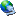Logged
EduardGender:Posts: 32
Reputation: 7.51
Rate Eduard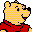I'm a llama!Re:Classic Texts: Bertrand Russell - Philosophy of Mathematical Logic (1911) « Reply #1 on: 2005-11-15 07:56:21 »From that still interesting classic,
Quote:
 To sum up, we have seen, in the first place, that mathematical logic has resolved the problems of infinity and continuity, and that it has made possible a solid philosophy of space, time, and motion. In the second place, we have seen that pure mathematics can be defined as the class of propositions which are expressed exclusively in terms of variables and logical constants, that is to say as the class of purely formal propositions. In the third place, we have seen that the possibility of mathematical knowledge refutes both empiricism and idealism, since it shows that human knowledge is not wholly deduced from facts of sense, but that a priori knowledge can by no means be explained in a subjective or psychological manner.
However, that class of propositions still seems mysterious...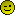Report to moderatorLogged

Insight?
 Jump to:Please select a destination: ----------------------------- General ----------------------------- => =>Evolution and Memetics =>FAQ =>Church Doctrine =>Science & Technology =>Society & Culture =>Arts & Entertainment =>Philosophy & Religion =>Humor & Satire =>Creative Endeavors =>Serious Business =>Virian Book Club =>Second Life =>Free For All =>Infections =>Suggestion Box =>Test Area ----------------------------- Mailing List ----------------------------- =>Virus 2006 =>Virus 2005 =>Virus 2004 =>Virus 2003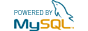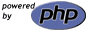Church of Virus BBS | Powered by YaBB SE © 2001-2002, YaBB SE Dev Team. All Rights Reserved. Please support the CoV.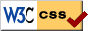RSS feed